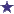# Convert 418MG to Grams Using the Ratio-Proportion Method

by Seacy
(Durham, NC )Ratio-Proportion Method

Convert the following units by using the ratio-proportion method.

418 mg = _____ g

### Comments for Convert 418MG to Grams Using the Ratio-Proportion Method

Average RatingRating418mg=0.418gm by: David How many milligrams in 1 gram? 1000 Multiply 418mg by 1gm/1000mg and you get: 0.418gm